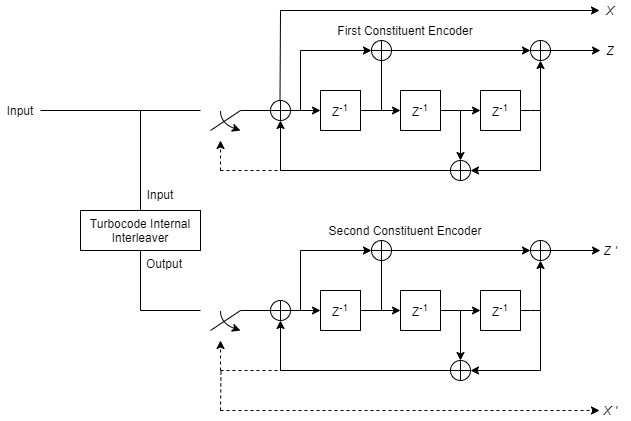# Turbo Encoder

Encode binary data using parallel concatenated encoding scheme

## Library

Convolutional sublibrary of Error Detection and Correction

•## Description

The Turbo Encoder block encodes a binary input signal using a parallel concatenated coding scheme. This coding scheme employs two identical convolutional encoders and one internal interleaver. Each constituent encoder is independently terminated by tail bits.

### Block Diagram of Parallel Concatenated Convolutional CodeThe previous block diagram illustrates that the output of the Turbo Encoder block consists of the systematic and parity bits streams of the first encoder, and only the parity bit streams of the second encoder.

For a rate one-half constituent encoder, the block interlaces the three streams and multiplexes the tail bits to the end of the encoded data streams.

For more information about tail bits, see the terminate Operation mode on the Convolutional Encoder block reference page.

### Dimensions

This block accepts an L-by-1 column vector input signal and outputs an M-by-1 column vector signal. For a given trellis, M and L are related by:

`$M=\text{\hspace{0.17em}}L\cdot \left(2\cdot n-1\right)+\text{\hspace{0.17em}}\text{​}2\cdot numTails$`

and

`$L=\text{\hspace{0.17em}}\frac{\left(M-2\cdot numTails\right)}{\left(2\cdot n-1\right)}$`

where

L = encoder input length

M = encoder output length

n = log2(trellis.NumOutputSymbols), for a rate 1/2 trellis, n = 2

numTails = log2(trellis.numStates) * n

### Encoder Schematic for Rate 1/3 Turbo Code ExampleThe previous schematic shows the encoder configuration for a trellis specified by the default value of the Trellis structure parameter, `poly2trellis(4, [13 15], 13)`. For an input vector length of 64 bits, the output of the encoder block is 204 bits. The first 192 bits correspond to the three 64 bit streams (systematic (Xk) and parity (Zk) bit streams from the first encoder and the parity (Zk) bit stream of the second encoder), interlaced as per Xk, Zk, Zk. The last 12 bits correspond to the tail bits from the two encoders, when the switches are in the lower position corresponding to the dashed lines. The first group of six bits (three systematic bits and three parity bits) are the output tail bits from the first constituent encoder. The second group of six bits (three systematic bits and three parity bits) are the output tail bits from the second constituent encoder.

Due to the tail bits, the encoder output code rate is slightly less than 1/3.

## Parameters

Trellis structure

Trellis structure of constituent convolutional code.

Specify the trellis as a MATLAB® structure that contains the trellis description of the constituent convolutional code. Alternatively, use the `poly2trellis` function to create a custom trellis using the constraint length, code generator (octal), and feedback connections (octal).

This block supports only rate 1-by-N trellises where N is an integer.

The default structure is the result of poly2trellis(4, [13 15], 13).

Source of interleaver indices

Specify the source of the interleaver indices as `Property` or ```Input port```.

When you set this parameter to `Property`, the block uses the Interleaver indices parameter to specify the interleaver indices.

When you set this parameter to `Input port`, the block uses the secondary input port, IntrInd, to specify the interleaver indices.

Interleaver indices

Specify the mapping that the block uses to permute the input bits as a column vector of integers. The default is `(64:-1:1).'`. This mapping is a vector with the number of elements equal to the length, L, of the input signal. Each element must be an integer between 1 and L, with no repeated values.

Simulate using

Specify if the block simulates using ```Code generation``` or ```Interpreted execution```. The default is ```Interpreted execution```.

## Supported Data Type

PortSupported Data Types
In
• Double

• Single

• Fixed-point

Out
• Double

• Single

• Fixed-point

## Examples

For an example that uses the Turbo Encoder and Turbo Decoder blocks, see the Parallel Concatenated Convolutional Coding: Turbo Codes example.

Turbo Decoder

## References

 Berrou, C., A. Glavieux, and P. Thitimajshima. "Near Shannon limit error correcting coding and decoding: turbo codes,” Proceedings of the IEEE International Conference on Communications, Geneva, Switzerland, May 1993, pp. 1064–1070.

 Benedetto, S., G. Montorsi, D. Divsalar, and F. Pollara. “ Soft-Input Soft-Output Maximum A Posterior (MAP) Module to Decode Parallel and Serial Concatenated Codes,”Jet Propulsion Lab TDA Progress Report, Vol. 42–27, Nov. 1996.

 Schlegel, Christian B. and Lance C. Perez. Trellis and Turbo Coding, IEEE Press, 2004.

 3GPP TS 36.212 v9.0.0, 3rd Generation partnership project; Technical specification group radio access network; Evolved Universal Terrestrial Radio Access (E-UTRA); Multiplexing and channel coding (release 9), 2009-12.

## Extended Capabilities

### Objects

Introduced in R2011b